# NCERT Solutions for Class 6 Maths Integers Chapter 6 Exercise 6.2 and 6.3

## NCERT Solutions for Class 6 Maths Chapter 6 Exercise 6.2 and 6.3

In this page we have NCERT Solutions for Class 6 Maths Integers Chapter 6 Exercise 6.2 and 6.3. Hope you like them and do not forget to like , social share and comment at the end of the page.
• When we have the same sign, add and put the same sign.
• When one positive and one negative integers are added we subtract them as whole numbers by considering the numbers without their sign and then put the sign of the bigger number with the subtraction obtained
• The subtraction of an integer is the same as the addition of its additive inverse.

### Exercise 6.2

Question 1
Using the number line write the integer which is:
(a) 3 more than 5
(b) 5 more than −5
(c) 6 less than 2
(d) 3 less than −2
More means moving right on the number line and less mean moving left on the Number line
a)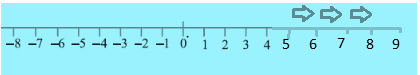b)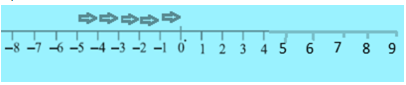c)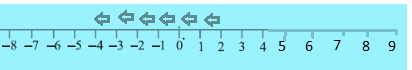d)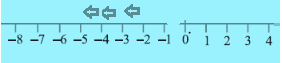Question 2
Use number line and add the following integers:
(a) $9 + (- 6)$
(b) $5 + (-11)$
(c) $(- 1) + (- 7)$
(d) $(- 5) + 10$
(e) $(- 1) + (- 2) + (- 3)$
(f) $(-2) + 8 + (- 4)$
Two important things to remember
When we add positive integer, we move to the right
When we add negative integer, we move to the right
a) $9 + (- 6)$
We start with number 9 and move to left six steps and we reach the number 3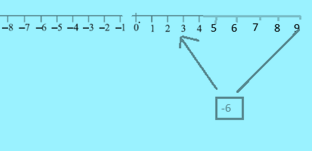b) $5 + (-11)$
We start with number 5 and move to left 11 steps and we reach the number -6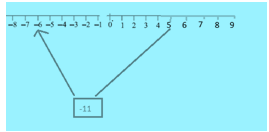c) $(- 1) + (- 7)$
We start with number -1 and move to left 7 steps and we reach the number -8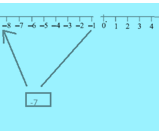d) $(- 5) + 10$
We start with number -5 and move to right 10 steps and we reach the number 5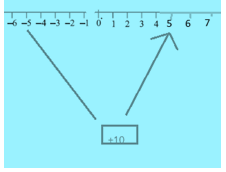e) $(-1) + (- 2) + (-3)$
We start with number -1 and move to left 2 steps and we reach the number -3. Then again we move to left 3 steps and we reach finally -6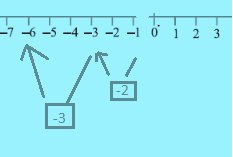f) $(- 2) + 8 + (-4)$
We start with number -2 and move to right 8 steps and we reach the number 6. Then again we move to left 4 steps and we reach finally 2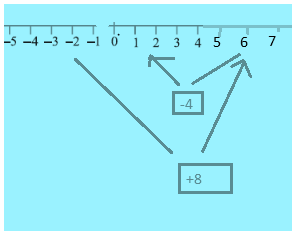Question 3
(a) $11 + (- 7)$
(b) $(- 13) + (+18)$
(c) $(- 10) + (+19)$
(d) $(- 250) + (+ 150)$
(e) $(- 380) + (- 270)$
(f) $(- 217) + (- 100)$
We have to take important note of this principle
1) When one positive and one negative integers are added, we subtract the smaller number from bigger number and put the sign of the bigger integer. The bigger integer is decided by ignoring the signs of the integers
For example, $(+11) + (-5) = + 6$ and $(-12) + (+ 1) = - 11$
2) When two negative integers are added, we add both the number and put the negative sign on the number=
For example, $(-11) + (-5) = -16$ and $(-12) + (- 1) = -13$
With this in mind, lets solve all the questions given above
 a) $11 + (- 7)$ Subtracting 7 from 11= 4 +4 b) $(- 13) + (+18)$ Subtracting 13 from 18= 5 +5 c) $(- 10) + (+19)$ Subtracting 10 from 19= 9 +9 d) $(- 250) + (+ 150)$ Subtracting 150 from 250= 100 -100 e) $(- 380) + (- 270)$ Adding 380 and 270=650 -650 f) $(- 217) + (- 100)$ Adding 217 and 100=317 -317

Question 4
Find the sum
(a) 137 and −354
(b) − 52 and 52
(c) − 312, 39 and 192
(d) − 50, − 200 and 300
We have to take important note of this principle
1) When one positive and one negative integers are added, we subtract the smaller number from bigger number and put the sign of the bigger integer. The bigger integer is decided by ignoring the signs of the integers
For example, (+11) + (–5) = + 6 and (–12) + (+ 1) = – 11
2) When two negative integers are added, we add both the number and put the negative sign on the number=
For example, (-11) + (–5) = -16 and (–12) + (- 1) = – 13
Also when three or more numbers are present, we start with first pair of 2 number, the answer from first pair is added to the third Number and it goes on.
 a) 137 and −354 Subtracting 137 from 354= 217 -217 b) − 52 and 52 Subtracting 52 from 52= 0 0 c) − 312, 39 and 192 1) Taking number -312 and 39 first Subtracting 39 from 312= 273 -273 2) Taking the number -273 and 192 Subtracting 192 from 273= 81 -81 d) − 50, − 200 and 300 1) Taking number -50 and -200 first Adding 50 and 200= 250 -250 2) Taking the number -250 and 300 Subtracting 250 from 300=50 50

Question 5
Find the sum:
(a) $(- 7) + (- 9) + 4 + 16$
(b) $(37) + (-2) + (- 65) + (- 8)$
a. $(- 7) + (- 9) + 4 + 16 = (-16) + (20) =4$
b. $(37) + (-2) + (- 65) + (- 8) = (37) + (-75) = (-38)$

## Try these NCERT book solutions

(a) (- 11) + (- 12)
(b) (+ 10) + (+ 4)
(c) (- 32) + (- 25)
(d) (+ 23) + (+ 40)
a.-23
b. 14
c. -57
d. 63

Question 2. Find the solution of the following:
(a) (-7) + (+ 8)
(b) (-9) + (+13)
(c) (+ 7) + (- 10)
(d) (+12) + (- 7)
a. 1
b. 4
c. -3
d. 5

Question 3. Find the solution of the following without using number line :
(a) (+ 7) + (- 11)
(b) (- 13) + (+ 10)
(c) (- 7) + (+ 9)
(d) (+ 10) + (-5)
Make five such questions and solve them.
a. -4
b. -3
c. -2
d. 5

### Exercise 6.3

Question 1
Find:
(a) $35 - (20)$
(b) $72 - (90)$
(c) $(- 15) - (- 18)$
(d) $(-20) - (13)$
(e) $23 - (- 12)$
(f) $(- 32) - (- 40)$

To subtract two integers, we add the additive inverse of the integer that is being subtracted.
So 2 -5 = 2+ (-5) as -5 is additive inverse of 5
 a) 35 − (20) = 35+ (-20) Subtract 35 and 20 and put the sign of the bigger number 35, which is positive 15 b) 72 − (90) 72+(-90) Subtract 72 and 90 and put the sign of the bigger number 90, which is negative -18 c) (− 15) − (− 18) = (-15) + (18) As additive inverse of -18 is 18 Subtract 15 and 18 and put the sign of the bigger number 18, which is negative 3 d) (− 20) − (13) = (-20) + (13) Subtract 20 and 13 and put the sign of the bigger number 20, which is negative -7 e) 23 − (− 12) =23+12 Adding the numbers 23 and 12= 35 f) (− 32) − (− 40) = (-32) + (40) Subtract 32 and 40 and put the sign of the bigger number 40, which is negative 8

Question 2
Fill in the blanks with >, < or = sign.
(a) (− 3) + (− 6) _____ (− 3) − (− 6)
(b) (− 21) − (− 10) _____ (− 31) + (− 11)
(c) 45 − (− 11) _____ 57 + (− 4)
(d) (− 25) − (− 42) _____ (− 42) − (−25)
We need to use the same addition and subtraction rules on both sides and then perform comparison
 S.no Question LHS RHS Answer a) (− 3) + (− 6) _____ (− 3) − (− 6) -9 3 < b) (− 21) − (− 10) _____ (− 31) + (− 11) -11 -32 > c) 45 − (− 11) _____ 57 + (− 4) 55 53 > d) (− 25) − (− 42) _____ (− 42) − (−25) 17 -17 >

Question 3
Fill in the blanks.
(a) (- 8) + _____ = 0
(b) 13 + _____ = 0
(c) 12 + (− 12) = _____
(d) (− 4) + _____ = − 12
(e) _____ − 15 = − 10
 a) (− 8) + _____ = 0 8 b) 13 + _____ = 0 -13 c) 12 + (− 12) = ___ 0 d) (− 4) + _____ = − 12 -8 e) _____ − 15 = − 10 5

Question 4
Find:
(a) $(- 7) - 8 - (-25)$
(b) $(-13) + 32 - 8 - 1$
(c) $(- 7) + (- 8) + (- 90)$
(d) $50 - (- 40) - (- 2)$
(a)$(- 7) - 8 - (-25) = (-15) - (-25) = (-15) + 25 = 10$
(b)$(-13) + 32 - 8 - 1 = 19 - 8 - 1 = 11 - 1 = 10$
(c) $(- 7) + (- 8) + (- 90) = (-15) + (-90) = (-105)$
(d) $50 - (- 40) - (- 2) = 50 + 40 - (-2) = 90 - (-2) = 90 + 2 = 92$### Practice Question

Question 1 What is $\frac {1}{2} + \frac {3}{4}$ ?
A)$\frac {5}{4}$
B)$\frac {1}{4}$
C)$1$
D)$\frac {4}{5}$
Question 2 Pinhole camera produces an ?
A)An erect and small image
B)an Inverted and small image
C)An inverted and enlarged image
D)None of the above